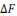As shown in a previous post, for samples taken from a zero mean i.i.d noise signal, the expected power of the k’th DFT coefficient is given byAs discussed in that post, when plotting the power of the k’th coefficient as a function of frequency, the “noise floor” will decrease as N increases — even though the noise power is the same regardless of the value of N chosen.

In situations where it is desirable to avoid this dependency, we can use the DFT to approximate the power spectral density. To see how this is done, consider that the frequency spacingbetween DFT coefficients depends on the sampling frequency, Fs, and the number of samples N as follows:The power of the k’th coefficient represents the power of all the energy in a bin of widthTherefore, the power spectral density in Watts/Hz at the frequency corresponding to the k’th coefficient is given by the following ratioNote that this value is independent of N. Furthermore, the DFT gives a useful representation of the spectrum over the range… so we can get the total power of the signal by multiplying the bandwidth Fs times the power spectral density to get, as expected,All of this means that defining the noise floor to beWatts/Hz

is a good approach. The noise variance is easily measured in the time domain in most systems by just collecting a large number of samples with no input signal applied and then computing the variance of the samples. The sampling rate and the resistor at which the noise samples are collected are normally fixed.

Furthermore, when analyzing data signals in the frequency domain, it should be clear that a spectrum program that plots Watts/Hz is a good choice. The noise floor will appear constant from plot to plot regardless of how many data samples are transformed, and signals will rise above and fall below the noise floor depending on their strength.

Mike Perkins, Ph.D., is a managing partner of Cardinal Peak and an expert in algorithm development for video and signal processing applications.